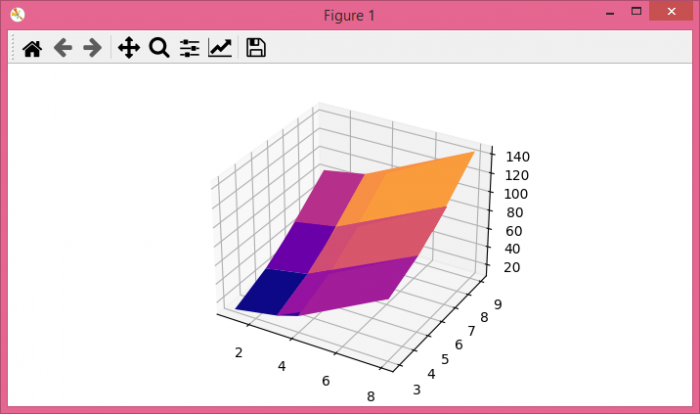# Plotting a 3D surface from a list of tuples in matplotlib?

To plot a 3D surface from a list of tuples in matplotlib, we can take the following steps.

## Steps

• Set the figure size and adjust the padding between and around the subplots.

• Make a list of tuples.

• Get the x, y and z data points from the list of tuples.

• Return the coordinate matrices from the coordinate vectors.

• Get the h data points for the surface plot.

• Create a new figure or activate an existing figure.

• Get the current axis, 3d, of the figure.

• Create a surface plot.

• To display the figure, use Show() method.

## Example

import numpy as np
from matplotlib import pyplot as plt

plt.rcParams["figure.figsize"] = [7.00, 3.50]
plt.rcParams["figure.autolayout"] = True

# List of tuples
data = [(1, 3, 2), (3, 5, 2), (4, 7, 4), (8, 7, 4),
(3, 6, 1), (3, 9, 0), (3, 9, 0)]

# Data points from the list of tuples
x, y, z = zip(*data)

x, y = np.meshgrid(x, y)

h = x ** 2 + y ** 2

fig = plt.figure()

# Get the current axis
ax = fig.gca(projection='3d')

# Surface plot
ax.plot_surface(x, y, h, cmap='plasma')

plt.show()


## Output

It will produce the following output −